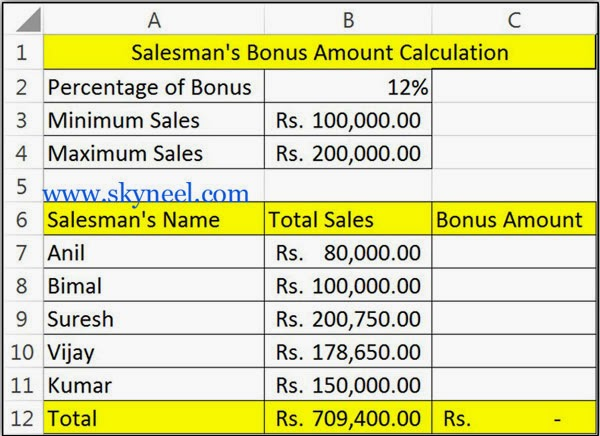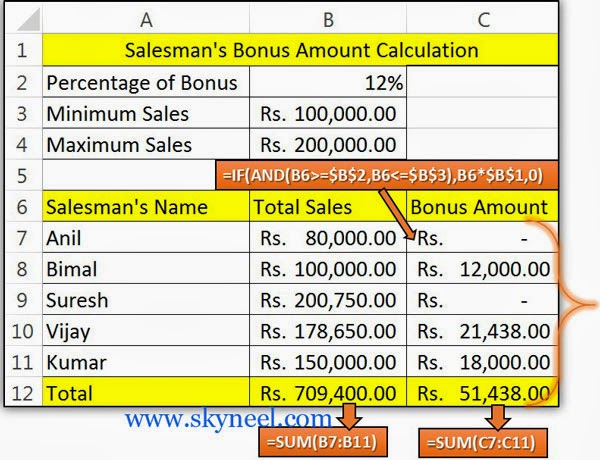# IF Statement with And Function in MS Excel

How to use IF Statement with And Function in MS Excel. This is a step by step easy guide with images.

Microsoft Excel IF( ) function is very popular and widely used a logical category function. This function returns first value if given condition is evaluates to TRUE, or second value if it evaluates to FALSE, while AND function returns TRUE if all its arguments are TRUE, returns FALSE if one or more argument is FALSE. So here is a guide for how to use IF Statement with And Function in MS Excel.

## How to use IF Statement with And Function:

IF Function ()

It is one of the most useful logical function of MS Excel. This function is used to check given condition is true or false. If the given condition is true, then function will return one value, if the condition is false, the function will do something else.

Syntax IF function:

IF(logic test, value if true, value if false) The logic test compare between given two values by using any comparison operator. The body part of this function are also known as arguments.

AND () Function
Returns TRUE, if all given values or arguments are TRUE. Returns FALSE if any single or multiple arguments are FALSE.

Syntax AND function

AND(logical1,logical2, …)
Logical1, logical2, logical3, …   are 1 to 255 conditions.
If you are using AND with if function then if both condition are true then result will be true, if any one or both condition result is false then the result will be false.

Example:
=AND(5>3,5<10)               True
=AND(5>13,5<10)             False
=AND(5>3,5<1)                False
=AND(5>13,5<1)               False

e.g. If you want to give bonus to your salesman 12% of his total sales, if your sales is greater than or equal to 100000 and less than or equal to 200000. In that case you have to take following steps.

Step 1: You have to create following sheet as per given below to calculate bonus of salesman in your company.Step 2: Now take the cursor on cell C7 and type this formula =IF(AND(B7>=\$B\$3,B7<=\$B\$4),B7*\$B\$2,0) to calculate the bonus then press enter key.

Step 3: Now copy this formula in range C8:C11.Step 4: After applying formula you will get following resulted sheet.Note: It is compulsory that you have to change cell reference type from relative cell (B2, B3, and B4) in  Absolute cell reference (\$B\$2, \$B\$3 and \$B\$4) in this formula.

I hope you like this if statement with and function guide from which you will be able to easily use if statement with and function without any extra efforts. If feel any problem in this guide then please use comment box. Thanks to all.